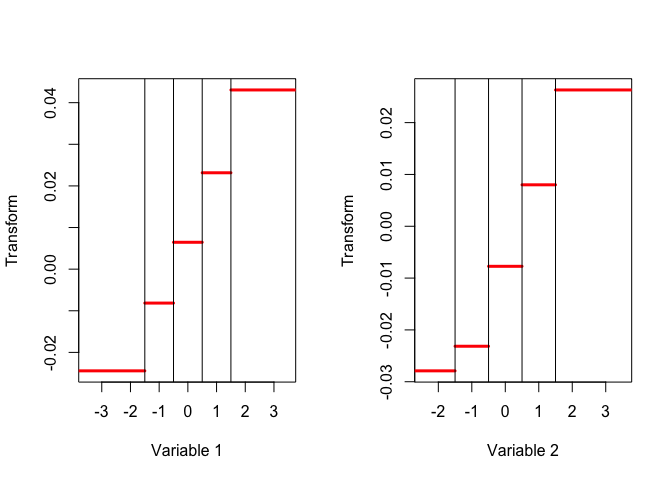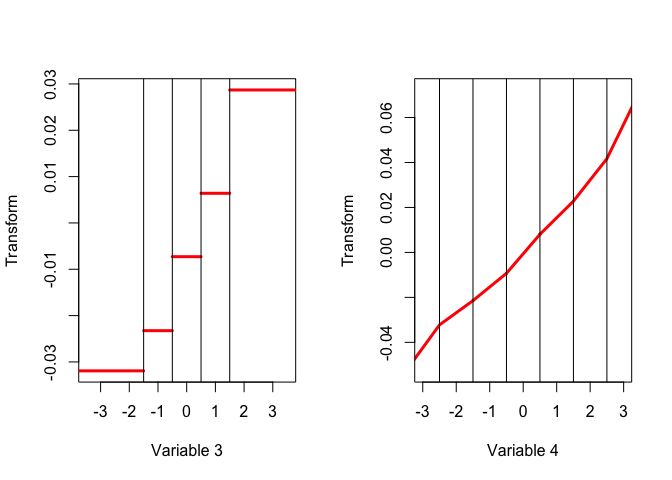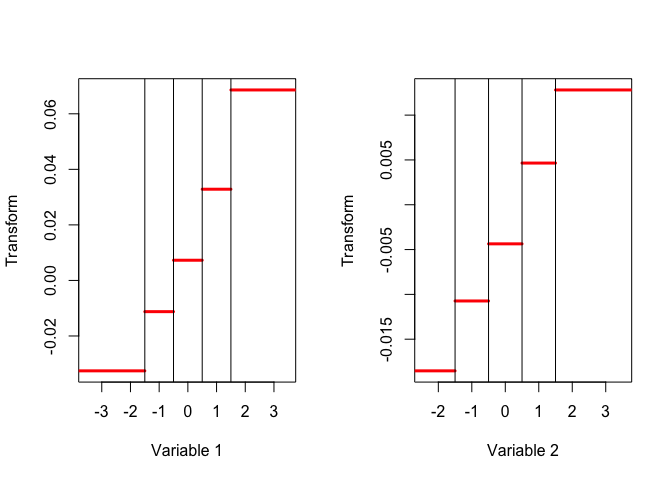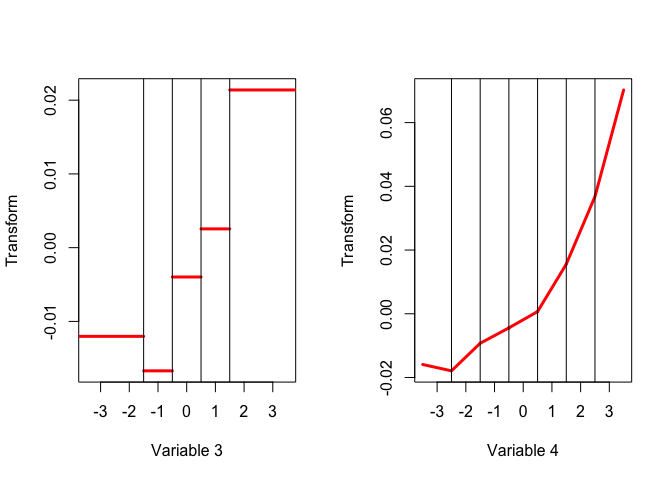Note: This is a working paper which will be expanded/updated frequently. One way to think about this paper is as an update of De Leeuw (1982), using more modern computing and reporting machinery. The directory deleeuwpdx.net/pubfolders/burt has a pdf copy of this article, the complete Rmd file with all code chunks, and R and C files with the code.

# 1 Basic Theory

## 1.1 Definition

Suppose $$X_1,\cdots,X_m$$ are data matrices, where $$X_j$$ is $$n\times k_j$$. Define $$X:=\begin{bmatrix}X_1\mid\cdots\mid X_m\end{bmatrix}$$ and $$C:=X'X$$. The matrix $$C$$ has $$k_j\times k_\ell$$ submatrices $$C_{j\ell}=X_j'X_\ell^{\ }$$. Also define $$D$$ as the direct sum $$D:=D_1\oplus\cdots\oplus D_m$$, where $$D_j:=C_{jj}$$. From now on we suppose that all $$D_j$$ are non-singular.

For $$m=3$$, for example, we have $C=\begin{bmatrix} C_{11}&C_{12}&C_{13}\\ C_{21}&C_{22}&C_{23}\\ C_{31}&C_{32}&C_{33} \end{bmatrix},$ and $D=\begin{bmatrix} D_1&0&0\\ 0&D_2&0\\ 0&0&D_3 \end{bmatrix}.$ Multiset Canonical Correlation Analysis (MCCA) finds one or more solutions of the generalized eigen-equation $CY=mDY\Lambda\tag{1},$ with the generalized eigenvectors normalized by $$Y'DY=I$$. Also see Tenenhaus and Tenenhaus (2011) and Van der Velden and Takane (2012).

Here is a small artificial example with three matrices. It shows uses the function listTable(), which constructs $$C$$ and $$D$$ from a list of matrices.

set.seed (12345)
x1 <- matrix (rnorm (300), 100, 3)
x2 <- matrix (rnorm (200), 100, 2)
x3 <- matrix (rnorm (300), 100, 3)
x <- list (x1, x2, x3)
f <- listTable (x)
f$c ## [,1] [,2] [,3] [,4] [,5] ## [1,] 1.000000000 0.10420973 -0.04320370 0.14055727 0.008395492 ## [2,] 0.104209727 1.00000000 -0.12665709 0.15100755 -0.154767884 ## [3,] -0.043203698 -0.12665709 1.00000000 -0.29195984 0.032050873 ## [4,] 0.140557275 0.15100755 -0.29195984 1.00000000 -0.127169849 ## [5,] 0.008395492 -0.15476788 0.03205087 -0.12716985 1.000000000 ## [6,] 0.043660968 0.10532711 0.03134388 0.08336952 0.137429305 ## [7,] -0.046180125 -0.08712887 -0.07733189 -0.06088433 -0.180728816 ## [8,] 0.121063405 0.10056221 -0.07985951 0.01814852 -0.282629632 ## [,6] [,7] [,8] ## [1,] 0.04366097 -0.04618013 0.12106341 ## [2,] 0.10532711 -0.08712887 0.10056221 ## [3,] 0.03134388 -0.07733189 -0.07985951 ## [4,] 0.08336952 -0.06088433 0.01814852 ## [5,] 0.13742930 -0.18072882 -0.28262963 ## [6,] 1.00000000 0.00278226 -0.18147091 ## [7,] 0.00278226 1.00000000 0.02598524 ## [8,] -0.18147091 0.02598524 1.00000000 f$d
##            [,1]       [,2]       [,3]       [,4]       [,5]        [,6]
## [1,]  1.0000000  0.1042097 -0.0432037  0.0000000  0.0000000  0.00000000
## [2,]  0.1042097  1.0000000 -0.1266571  0.0000000  0.0000000  0.00000000
## [3,] -0.0432037 -0.1266571  1.0000000  0.0000000  0.0000000  0.00000000
## [4,]  0.0000000  0.0000000  0.0000000  1.0000000 -0.1271698  0.00000000
## [5,]  0.0000000  0.0000000  0.0000000 -0.1271698  1.0000000  0.00000000
## [6,]  0.0000000  0.0000000  0.0000000  0.0000000  0.0000000  1.00000000
## [7,]  0.0000000  0.0000000  0.0000000  0.0000000  0.0000000  0.00278226
## [8,]  0.0000000  0.0000000  0.0000000  0.0000000  0.0000000 -0.18147091
##            [,7]        [,8]
## [1,] 0.00000000  0.00000000
## [2,] 0.00000000  0.00000000
## [3,] 0.00000000  0.00000000
## [4,] 0.00000000  0.00000000
## [5,] 0.00000000  0.00000000
## [6,] 0.00278226 -0.18147091
## [7,] 1.00000000  0.02598524
## [8,] 0.02598524  1.00000000

The listTable() function has some additional arguments, which can be used to optionally center, standardize, or orthonormalize the matrices in the list.

formals("listTable")
## $x ## ## ##$center
##  FALSE
##
## $standardize ##  FALSE ## ##$orthonormalize
##  FALSE

## 1.2 MCCA Eigenvalues

All eigenvalues of equation (1) satisfy $$0\leq\lambda\leq 1$$. Clearly $$m\lambda_s=y'Cy$$, which shows that $$\lambda\geq 0$$ and $$\lambda=0$$ if and only if $$Cy=0$$ if and only if $$Xy=0$$. The number of zero eigenvalues of (1) is consequently the nullity of $$X$$. To show that $$\lambda\leq 1$$ observe that $\sum_{j=1}^m(X_jy_j-\frac{1}{m}\sum_{j=1}^mX_jy_j)'(X_jy_j-\frac{1}{m}\sum_{j=1}^mX_jy_j)\geq 0,$ which can be written as $y'Dy-\frac{1}{m}y'Cy\geq 0.$ This proves $$\lambda\leq 1$$ and $$\lambda=1$$ if and only if all $$X_jy_j$$ are equal.

The following chunk computes the eigenvalues in our small example with three matrices.

library (geigen)
dim(f$c) ##  8 8 dim(f$d)
##  8 8
#h <- geigen (f$c / 3, f$d, s = TRUE)
#rev (h$values) Define $$Z$$ as $$\begin{bmatrix}X_1D_1^{-\frac12}\mid\cdots\mid X_mD_m^{-\frac12}\end{bmatrix}$$ and $$E:=Z'Z$$. Thus $$E_{j\ell}=D_j^{-\frac12}C_{j\ell}D_\ell^{-\frac12}$$ and $$E_{jj}=I$$ for all $$j$$. For $$m=3$$ we have $E=\begin{bmatrix} I&D_1^{-\frac12}C_{12}D_2^{-\frac12}&D_1^{-\frac12}C_{13}D_3^{-\frac12}\\ D_2^{-\frac12}C_{21}D_1^{-\frac12}&I&D_2^{-\frac12}C_{23}D_3^{-\frac12}\\ D_3^{-\frac12}C_{31}D_1^{-\frac12}&D_3^{-\frac12}C_{32}D_2^{-\frac12}&I \end{bmatrix}$ The eigenvalues of $$\frac{1}{m}E$$ are the same as those of (1). It follows that $\mathbf{tr}\ \Lambda=\frac{1}{m}\sum_{j=1}^m k_j.$ The sum of squares of the eigenvalues is $$\frac{1}{m^2}\mathbf{tr}\ E'E$$. The matrix $$D_j^{-\frac12}$$ can be the inverse of the symmetric square root of $$D_j$$, or the inverse of the triangular factor in the QR decomposition of $$X_j$$. In fact it can be any nonsingular $$T$$ such that $$T'D_jT=I$$. Solutions for $$T$$ differ only by a rotation matrix (a square orthonormal), and thus the choice of $$T$$ does not change the eigenvalues of $$E$$. The matrix $$E$$ can be created by using listTable() with argument orthonormal=TRUE. It uses the Gram-Schmidt code in J. De Leeuw (2015a) for the orthonormalization. f <- listTable (x, o = TRUE) f$c
##               [,1]          [,2]          [,3]          [,4]          [,5]
## [1,]  1.000000e+00 -2.081668e-17  5.551115e-17  1.809187e-01  2.137444e-02
## [2,] -2.081668e-17  1.000000e+00  4.510281e-17  1.377245e-01 -1.400175e-01
## [3,]  5.551115e-17  4.510281e-17  1.000000e+00 -2.713240e-01 -2.183805e-02
## [4,]  1.809187e-01  1.377245e-01 -2.713240e-01  1.000000e+00  8.651933e-17
## [5,]  2.137444e-02 -1.400175e-01 -2.183805e-02  8.651933e-17  1.000000e+00
## [6,]  5.531522e-02  1.021675e-01  4.425189e-02  9.408917e-02  1.479562e-01
## [7,] -4.646289e-02 -8.290192e-02 -9.053349e-02 -6.090152e-02 -1.905037e-01
## [8,]  1.735305e-01  1.107133e-01 -6.072594e-02  8.107887e-02 -2.557320e-01
##               [,6]          [,7]          [,8]
## [1,]  5.531522e-02 -4.646289e-02  1.735305e-01
## [2,]  1.021675e-01 -8.290192e-02  1.107133e-01
## [3,]  4.425189e-02 -9.053349e-02 -6.072594e-02
## [4,]  9.408917e-02 -6.090152e-02  8.107887e-02
## [5,]  1.479562e-01 -1.905037e-01 -2.557320e-01
## [6,]  1.000000e+00  3.469447e-17 -5.551115e-17
## [7,]  3.469447e-17  1.000000e+00  2.081668e-17
## [8,] -5.551115e-17  2.081668e-17  1.000000e+00
eigen (f$c / length (x), symmetric = TRUE, only.values = TRUE)$values
##  0.4967371 0.4448935 0.3852558 0.3440331 0.3295966 0.2575979 0.2217012
##  0.1868515

Matrices $$\tilde X'\tilde X$$ and $$\tilde X\tilde X'$$ have the same non-zero eigenvalues, the squares of the singular values of $$\tilde X$$. Thus the non-zero $$\lambda_s$$ are also the non-zero eigenvalues of $P_\bullet:=\frac{1}{m}\sum_{j=1}^m P_j,$ where $$P_j:=X_j(X_j'X_j)^{-1}X_j'$$. Note that $$P_j$$ does not change if $$X_j$$ is replaced by $$X_jT$$ with $$T$$ nonsingular.

p <- tcrossprod (f$g) / 3 eigen (p, symmetric = TRUE, only.values = TRUE)$values[1:8]
##  0.4967371 0.4448935 0.3852558 0.3440331 0.3295966 0.2575979 0.2217012
##  0.1868515

## 1.3 Least Squares Loss Function

For computational purposes, especially if we incorporate optimal scaling in MCCA as in Gifi (1990), it is convenient to formulate the technique as minimization of a least squares loss function. This formulation originates with Carroll (1968). The loss function is $\sigma(H;Y_1,\cdots,Y_m)=\frac{1}{m}\sum_{j=1}^m\mathbf{tr}\ (H-X_jY_j)'(H-X_jY_j),$ which we minimize over the $$Y_j$$ and over all $$H$$ satisfying $$H'H=I$$. We choose the dimensionality $$p$$. Since $\min_{Y_1,\cdots,Y_m}\sigma(H;Y_1,\cdots,Y_m)=\frac{1}{m}\sum_{j=1}^m\mathbf{tr}\ H'(I-P_j)H,$ we see that the minimizing $$H$$ are the eigenvectors corresponding to the $$p$$ largest eigenvalues of $$P_\bullet$$, and $\min_{H'H=I}\min_{Y_1,\cdots,Y_m}\sigma(H;Y_1,\cdots,Y_m)=\sum_{s=p+1}^m(1-\lambda_s).$ For the corresponding optimum $$Y_j$$ we find $$D_j^\frac12 Y_j=Z_j'H$$, and thus $$Y'DY=mH'P_\bullet H=m\Lambda$$. We have $$CY=mDY\Lambda$$, but the columns of $$Y$$ are normalized to the size of the eigenvalues.

# 2 Multiple Correspondence Analysis

## 2.1 The Burt Table

In the special case in which the $$X_j$$ are indicator matrices, i.e. non-negative matrices with rows that add up to one, our MCCA technique becomes Multiple Correspondence Analysis (MCA). In this case the matrix $$C$$ is called the Burt Table. For a detailed discussion, see Greenacre and Blasius (2006) and specifically De Leeuw (2006).

Two different cases can be distinguished. The indicator matrices can be binary, also known as crisp, in which case they obviously have a single nonzero element equal to one in each row, or they can be fuzzy indicators, discussed in detail in Van Rijckevorsel and De Leeuw (1988). The burtTable() function handles both cases by using the B-spline code in J. De Leeuw (2015). The number of nonzero elements in each row of the indicator matrix is determined by the degree of the spline, with degree zero defining the crisp indicator. The number of columns of $$X_j$$ is determined, in addition, by the number of interior knots of the spline. There is a special provision to generate crisp indicators from categorical, possibly non-numerical, variables by setting the degree equal to a negative number and by ignoring the knots argument.

In the following example we generate a sample from a four-variable standard multinormal with correlations $$\frac12$$, rounded to integers. All four variables generate indicators by using knots at $$-1.5, -.5, +.5$$ and $$+1.5$$, the first three have spline degree zero and the fourth has spline degree two Thus there are three crisp indicators and one fuzzy indicator.

set.seed (12345)
x <- ceiling ( (matrix (rnorm (4000), 1000, 4) + rnorm (1000)) / sqrt (2))
x <- center (x)
n <- c(-1.5, -.5, .5, 1.5)
k <- list (n, n, n, n)
fp <- burtTable (x, c (0, 0, 0, 2), k)
dp <- blockSelect (fp$c, fp$ord)
hp <- geigen (fp$c / 4, dp, symmetric = TRUE) rev (hp$values[-c(1,2,3)])[-1]
##   0.5970850 0.4037270 0.3107367 0.2823285 0.2720878 0.2638761 0.2562212
##   0.2477486 0.2387625 0.2340783 0.2222054 0.2105898 0.2042858 0.1867432
##  0.1765546 0.1399286 0.1279515 0.1250894Figure 1: MCA - PCA Option

In MCA we typically use the indicator matrices to define one $$X_j$$ each. But in the Gifi system (Gifi 1990, Michailidis and De Leeuw (1998), De Leeuw and Mair (2009), J. De Leeuw (2015b)) we can group the indicators into sets of variables to emulate other forms of multivariate analysis. In the next analysis we use two blocks, one consisting of the first variable and one of the remaining three. This makes the technique a form of regression analysis, where the first variable is predicted from the rest.

fr <- burtTable (x, c (0, 0, 0, 2), k, center = TRUE)
dr <- blockSelect (fr$c, c(fr$ord, sum (fr$ord[-1]))) hr <- geigen (fr$c / 2, dr, symmetric = TRUE)
sort (2 * hr$values - 1, decreasing = TRUE)[1:4] ##  0.5916795 0.3531064 0.1975003 0.1061619Figure 2: MCA - Regression Option ## 2.2 Using Homogeneity The MCCA problem can be formulated as finding stationary values of $\lambda(y_1,\cdots,y_m)=\sum_{j=1}^m\sum_{\ell=1}^m y_j'C_{j\ell}y_\ell$ over the $$y_j$$ satisfying $$\sum_{j=1}^m y_j'D_jy_j=m$$. Alternatively we can look for stationary values of $\lambda(\alpha_1,\cdots,\alpha_m,\eta_1,\cdots,\eta_m)=\sum_{j=1}^m\sum_{\ell=1}^m \alpha_j\alpha_\ell\eta_j'C_{j\ell}\eta_\ell$ over $$\alpha_j$$ satisfying $$\sum_{j=1}^m\alpha_j^2=m$$ and $$\eta_j$$ satisfying $$\eta_j'D_j\eta_j=1$$ for all $$j$$. If we define $$R(\eta_1,\cdots,\eta_m)$$ with elements $$r(\eta_1,\cdots,\eta_m)_{j\ell}=\eta_j'C_{j\ell}\eta_\ell$$ then we want stationary values of $$\alpha'R(\eta_1,\cdots,\eta_m)\alpha$$ over $$\alpha'\alpha=m$$. ## 2.3 Induced Correlation Matrices print (r <- inducedR (fp$c, yp[, sum(fp$ord) - 1], fp$ord))
##           [,1]      [,2]      [,3]      [,4]
## [1,] 1.0000000 0.4484230 0.4828116 0.4649239
## [2,] 0.4484230 1.0000000 0.4462666 0.4766192
## [3,] 0.4828116 0.4462666 1.0000000 0.4575092
## [4,] 0.4649239 0.4766192 0.4575092 1.0000000
eigen(r)$values / 4 ##  0.5970850 0.1433047 0.1307759 0.1288345 print (r <- inducedR (fp$c, yp[, sum(fp$ord) - 2], fp$ord))
##           [,1]      [,2]      [,3]      [,4]
## [1,] 1.0000000 0.1657850 0.2323857 0.2654327
## [2,] 0.1657850 1.0000000 0.2021524 0.1658702
## [3,] 0.2323857 0.2021524 1.0000000 0.1922082
## [4,] 0.2654327 0.1658702 0.1922082 1.0000000
eigen(r)$values / 4 ##  0.4037270 0.2170939 0.1980885 0.1810906 inducedR (fr$c, yr[,sum(fr$ord)], c(fr$ord, sum (fr$ord[-1]))) ## [,1] [,2] ## [1,] 1.0000000 0.5916795 ## [2,] 0.5916795 1.0000000 inducedR (fr$c, yr[,sum(fr$ord) - 1], c(fr$ord, sum (fr$ord[-1]))) ## [,1] [,2] ## [1,] 1.0000000 0.3531064 ## [2,] 0.3531064 1.0000000 ## 2.4 Binary Variables ## 2.5 On Being Normal # 3 Linked Singular Value Decompositions ## 3.1 Pairwise Canonical Correlation Analysis If we define the $$K\times K$$ matrix $$\Gamma$$ by replacing each $$E_{j\ell}$$ by the diagonal matrix $$\Gamma_{j\ell}$$ of the canonical correlations between $$X_j$$ and $$X_\ell$$, then $\sum_{s=1}^K \lambda_s^2=\frac{1}{m^2}\mathbf{tr}\ E'E=\frac{1}{m^2}\mathbf{tr}\ \Gamma'\Gamma.$ The canonical correlations are the singular values of the matrices $$E_{j\ell}$$. The matrix $$\Gamma$$ is as follows. ## [,1] [,2] [,3] [,4] [,5] [,6] ## [1,] 1.0000000 0.0000000 0.00000000 0.336463 0.00000000 0.2219902 ## [2,] 0.0000000 1.0000000 0.00000000 0.000000 0.14069690 0.0000000 ## [3,] 0.0000000 0.0000000 1.00000000 0.000000 0.00000000 0.0000000 ## [4,] 0.3364630 0.0000000 0.00000000 1.000000 0.00000000 0.3541990 ## [5,] 0.0000000 0.1406969 0.00000000 0.000000 1.00000000 0.0000000 ## [6,] 0.2219902 0.0000000 0.00000000 0.354199 0.00000000 1.0000000 ## [7,] 0.0000000 0.1208259 0.00000000 0.000000 0.09977141 0.0000000 ## [8,] 0.0000000 0.0000000 0.02100453 0.000000 0.00000000 0.0000000 ## [,7] [,8] ## [1,] 0.00000000 0.00000000 ## [2,] 0.12082589 0.00000000 ## [3,] 0.00000000 0.02100453 ## [4,] 0.00000000 0.00000000 ## [5,] 0.09977141 0.00000000 ## [6,] 0.00000000 0.00000000 ## [7,] 1.00000000 0.00000000 ## [8,] 0.00000000 1.00000000 Indeed, both $$\mathbf{tr}\ E'E$$ and $$\mathbf{tr}\ \Gamma'\Gamma$$ are equal to 8.6654679. Although the sum and the sum of squares of the eigenvalues of $$E$$ and $$\Gamma$$ are the same, the individual eigenvalues differ. For $$\frac13\Gamma$$ they are ##  0.5372982 0.4138783 0.3403348 0.3263318 0.3009372 0.2851846 0.2595023 ##  0.2031994 We do see, however, that the two vectors of eigenvalues are quite close. This phenomenon is studied in more detail in a later section of the paper. For now we merely observe that we can permute rows and columns of $$\Gamma$$ so that it becomes the direct sum of three matrices $$R_1, R_2$$ and $$R_3$$. Think of $$\Gamma$$ as a supermatrices with nine submatrices, all diagonal. Matrix $$R_1$$ consists of all $$(1,1)$$ elements of the submatrices of $$\Gamma$$, matrix $$R_2$$ has the $$(2,2)$$ elements, and $$R_3$$ the $$(3,3)$$ elements. The utility function partPerm() does just that. print (rm <- kplPerm (gm, c(3,2,3))$pcp)
##           [,1]     [,2]      [,3]      [,4]       [,5]       [,6]
## [1,] 1.0000000 0.336463 0.2219902 0.0000000 0.00000000 0.00000000
## [2,] 0.3364630 1.000000 0.3541990 0.0000000 0.00000000 0.00000000
## [3,] 0.2219902 0.354199 1.0000000 0.0000000 0.00000000 0.00000000
## [4,] 0.0000000 0.000000 0.0000000 1.0000000 0.14069690 0.12082589
## [5,] 0.0000000 0.000000 0.0000000 0.1406969 1.00000000 0.09977141
## [6,] 0.0000000 0.000000 0.0000000 0.1208259 0.09977141 1.00000000
## [7,] 0.0000000 0.000000 0.0000000 0.0000000 0.00000000 0.00000000
## [8,] 0.0000000 0.000000 0.0000000 0.0000000 0.00000000 0.00000000
##            [,7]       [,8]
## [1,] 0.00000000 0.00000000
## [2,] 0.00000000 0.00000000
## [3,] 0.00000000 0.00000000
## [4,] 0.00000000 0.00000000
## [5,] 0.00000000 0.00000000
## [6,] 0.00000000 0.00000000
## [7,] 1.00000000 0.02100453
## [8,] 0.02100453 1.00000000

The eigenvalues of this rearranged matrix are the same as those of $$\Gamma$$, and they are the direct sum of the eigenvalues of $$R_1, R_2$$ and $$R_3$$. Or, to put is slightly differently, we can find the eigenvalues of $$\Gamma$$ by first permuting to block diagonal form and then finding the eigenvalues of the blocks. This gives the eigenvalues in the order determined by the block structure (ordered within blocks).

##           [,1]      [,2]      [,3]      [,4]      [,5]      [,6]      [,7]
## [1,] 0.5372982 0.0000000 0.0000000 0.0000000 0.0000000 0.0000000 0.0000000
## [2,] 0.0000000 0.2595023 0.0000000 0.0000000 0.0000000 0.0000000 0.0000000
## [3,] 0.0000000 0.0000000 0.2031994 0.0000000 0.0000000 0.0000000 0.0000000
## [4,] 0.0000000 0.0000000 0.0000000 0.4138783 0.0000000 0.0000000 0.0000000
## [5,] 0.0000000 0.0000000 0.0000000 0.0000000 0.3009372 0.0000000 0.0000000
## [6,] 0.0000000 0.0000000 0.0000000 0.0000000 0.0000000 0.2851846 0.0000000
## [7,] 0.0000000 0.0000000 0.0000000 0.0000000 0.0000000 0.0000000 0.3403348
## [8,] 0.0000000 0.0000000 0.0000000 0.0000000 0.0000000 0.0000000 0.0000000
##           [,8]
## [1,] 0.0000000
## [2,] 0.0000000
## [3,] 0.0000000
## [4,] 0.0000000
## [5,] 0.0000000
## [6,] 0.0000000
## [7,] 0.0000000
## [8,] 0.3263318

Using pairwise canonical correlations, followed by permutation to block diagonal form, cannot be recommended as a general MCCA technique. There is no obvious loss function that is minimized, and it is not clear how the canonical correlations in the different blocks should be ordered.

## 3.2 Two Sets of Variables

If there are only two sets the generalized eigenvalue problem for the Burt matrix becomes $\begin{bmatrix} D_1&C_{12}\\C_{21}&D_2 \end{bmatrix} \begin{bmatrix} a_1\\a_2 \end{bmatrix}=2\lambda\begin{bmatrix}D_1&0\\0&D_2\end{bmatrix}\begin{bmatrix} a_1\\a_2 \end{bmatrix},$ which we can rewrite as $\begin{split} C_{12}a_2&=(2\lambda-1)D_1a_1,\\ C_{21}a_1&=(2\lambda-1)D_2a_2, \end{split}$ from which we see that MCCA finds the canonical correlations between $$X_1$$ and $$X_2$$. We also see that at a solution $$a_1'D_1a_1=a_2'D_2a_2=\frac12$$, and the canonical correlations are given by $$\rho=2\lambda-1$$. See also Van der Velden (2012).

## 3.3 KPL Diagonalization

In the section on pairwise canonical correlation analysis we saw that permuting pairwise canonical correlations to block diagonal form can give a decent approximation of the eigenvalues of MCCA. Here we describe the nature of this approximation in more detail, following De Leeuw (1982), Bekker and De Leeuw (1988), and De Leeuw (1988), and using the algorithm in (???).

In MCCA we have $$A'CA=m\Lambda$$ and $$A'DA=I$$. Or, alternatively, $$K'EK=m\Lambda$$ with $$K'K=KK'=I$$, i.e. $$E=mK\Lambda K'$$. In CCA we have $$E_{j\ell}=K_{j\ell}\Gamma_{j\ell}L_{j\ell}'$$.

Let’s first look at the same MCA example as before, but now with all variable bases defined by splines of degree zero (i.e. all indicators are crisp). We first compute the eigenvalues and then the approximations.

set.seed (12345)
x <- round ( (matrix (rnorm (4000), 1000, 4) + rnorm (1000)) / sqrt (2))
f <- burtTable (x, c (0,0,0,0), list (c (-1.5, 1.5), c (-1.5, 1.5), c (-1.5, 1.5), c (-1.5, 1.5)))
e <- makeE (f$c, c(3, 3, 3, 3)) eigen (e)$values[1:9] / 4
##  1.0000000 0.4519489 0.3527894 0.2367481 0.2098061 0.2043497 0.1926123
##  0.1786254 0.1731200
h <- kplSVD (e, c(3, 3 ,3, 3))
## Iteration      1 ssq      13.10653878
## Iteration      2 ssq      13.10701043
## Iteration      3 ssq      13.10727417
## Iteration      4 ssq      13.10737974
## Iteration      5 ssq      13.10742536
## Iteration      6 ssq      13.10744491
## Iteration      7 ssq       13.1074533
## Iteration      8 ssq       13.1074569
## Iteration      9 ssq      13.10745844
## Iteration     10 ssq      13.10745911
p <- kplPerm (h$kek, c(3, 3, 3 ,3)) eigen (blockSelect (p$pcp, p$ord))$values[1:9] / 4
##  1.0000000 0.4518596 0.3525997 0.2361880 0.2093469 0.2018654 0.1933701
##  0.1811957 0.1735746
x <- list(x1, x2, x3)
e <- listTable (x, o = TRUE)$c eigen (e)$values / 3
##  0.4788789 0.4456476 0.3882282 0.3430650 0.3294195 0.2673813 0.2265314
##  0.1875147
h <- kplSVD (e, c(3, 2, 3))
## Iteration      1 ssq     0.3488537599
## Iteration      2 ssq     0.3488578851
## Iteration      3 ssq     0.3488578869
p <- kplPerm (h$kek, c(3, 2, 3)) eigen (blockSelect (p$pcp, p$ord))$values / 3
##  0.4522979 0.3935826 0.3459171 0.3386260 0.3382622 0.3284045 0.2605004
##  0.2090761

# 4 Appendix: Code

dyn.load("gs.so")

bsplineBasis <-
function (x, degree, innerknots, lowknot = min(x,innerknots) - 1e-6, highknot = max(x,innerknots) + 1e-6) {
innerknots <- unique (sort (innerknots))
knots <-
c(rep(lowknot, degree + 1), innerknots, rep(highknot, degree + 1))
n <- length (x)
m <- length (innerknots) + 2 * (degree + 1)
nf <- length (innerknots) + degree + 1
basis <- rep (0,  n * nf)
res <- .C(
"splinebasis", d = as.integer(degree),
n = as.integer(n), m = as.integer (m), x = as.double (x), knots = as.double (knots), basis = as.double(basis)
)
basis <- matrix (res$basis, n, nf) basis <- basis[,which(colSums(basis) > 0)] return (basis) } gs<-function(x) { n<-dim(x); m<-dim(x); q<-matrix(0,n,m); r<-matrix(0,m,m) qr<-.C("gsc",as.double(x),as.double(q),as.double(r),as.integer(dim(x)), as.integer(dim(x))) return(list(q=matrix(qr[],n,m),r=matrix(qr[],m,m))) } center <- function (x) { return (apply (x, 2, function (z) z - mean (z))) } standardize <- function (x) { return (apply (x, 2, function (z) z / sqrt (sum (z ^ 2)))) } listTable <- function (x, center = TRUE, standardize = TRUE, orthonormalize = FALSE) { n <- nrow (x[]) m <- length (x) g <- matrix (0, n, 0) l <- rep (0, m) for (j in 1:m) { h <- x[[j]] if (center) { h <- center (h) } if (standardize) { h <- standardize (h) } if (orthonormalize) { h <- gs (h)$q
}
g <- cbind (g, h)
l[j] <- ncol (h)
}
return (list(c = crossprod (g),  g = g, ord = l))
}

burtTable <- function (x, degrees = rep (-1, ncol (x)), knots = NULL, center = FALSE, standardize = FALSE, orthonormalize = FALSE) {
n <- nrow (x)
m <- ncol (x)
g <- matrix (0, n, 0)
l <- rep (0, m)
for (j in 1:m) {
z <- x[,j]
if (degrees[j] < 0) {
h <- ifelse (outer (z, unique (z), "=="), 1, 0)
}
else {
h <- bsplineBasis (z, degrees [j], knots [[j]])
}
if (center) {
h <- center (h)[, -1]
}
if (standardize) {
h <- standardize (h)
}
if (orthonormalize) {
h <- gs (h)$q } g <- cbind (g, h) l[j] <- ncol (h) } return (list(c = crossprod (g), g = g, ord = l)) } kplPerm <- function (cc, k) { kl<-unlist (sapply (k, function (i) 1:i)) p <- ifelse (outer (1:sum (k), order (kl), "=="), 1, 0) return (list (pcp = t(p) %*% cc %*% p, perm = p, ord = as.vector (table (kl)))) } makeE <- function (cc, k) { dd <- mInvSqrt (blockSelect (cc, k)) return (dd %*% cc %*% dd) } mInvSqrt <- function (x) { ex <- eigen (x) ew <- abs (ex$values)
ev <- ifelse (ew == 0, 0, 1 / sqrt (ew))
ey <- ex$vectors return (ey %*% (ev * t (ey))) } blockSelect <- function (cc, k) { l <- unlist (lapply (1:length (k), function(i) rep (i,k[i]))) return (cc * ifelse (outer (l, l, "=="), 1, 0)) } directSum <- function (x) { m <- length (x) nr <- sum (sapply (x, nrow)) nc <- sum (sapply (x, ncol)) z <- matrix (0, nr, nc) kr <- 0 kc <- 0 for (i in 1:m) { ir <- nrow (x[[i]]) ic <- ncol (x[[i]]) z[kr + (1:ir), kc + (1:ic)] <- x[[i]] kr <- kr + ir kc <- kc + ic } return (z) } kplSVD <- function (e, k, eps = 1e-6, itmax = 500, verbose = TRUE, vectors = TRUE) { m <- length (k) sk <- sum (k) ll <- kk <- ww <- diag (sk) itel <- 1 ossq <- 0 klw <- 1 + cumsum (c (0, k))[1:m] kup <- cumsum (k) ind <- lapply (1:m, function (i) klw[i]:kup[i]) for (i in 1:m) kk[ind[[i]],ind[[i]]] <- t (svd (e[ind[[i]], ])$u)
kek <- kk %*% e %*% t(kk)
for (i in 1:m) for (j in 1:m)
ww[ind[[i]],ind[[j]]] <- ifelse(outer(1:k[i],1:k[j],"=="),1,0)
repeat {
for (l in 1:m) {
if (k[l] == 2) next()
li <- ind[[l]]
for (i in (klw[l] + 1):(kup[l]-1)) for (j in (i+1):kup[l]) {
bi <- kek[i,-li]
bj <- kek[j,-li]
wi <- ww[i,-li]
wj <- ww[j,-li]
acc <- sum(wi*bi^2)+sum(wj*bj^2)
acs <- sum((wi-wj)*bi*bj)
ass <- sum(wi*bj^2)+sum(wj*bi^2)
u <- eigen(matrix(c(acc,acs,acs,ass),2,2))\$vectors[,1]
c <- u
s <- u
kek[-li,i] <- kek[i,-li] <- c*bi+s*bj
kek[-li,j] <- kek[j,-li] <- c*bj-s*bi
if (vectors) {
ki <- kk[i,li]; kj <- kk[j,li]
kk[i,li] <- c*ki+s*kj
kk[j,li] <- c*kj-s*ki
}
}
}
nssq <- sum (ww * kek ^ 2) - sum (diag (kek) ^ 2)
if (verbose)
cat("Iteration ",formatC(itel,digits=4),"ssq ",formatC(nssq,digits=10,width=15),"\n")
if (((nssq - ossq) < eps) || (itel == itmax)) break()
itel <- itel + 1
ossq <- nssq
}
return(list(kek = kek, kk = kk, itel = itel, ssq = nssq))
}

inducedR <- function (c, y, k) {
m <- length (k)
l <- unlist (lapply (1:m, function(i) rep (i,k[i])))
g <- ifelse (outer (l, 1:m, "=="), 1, 0)
s <- g * matrix (y, length(y), m)
r <- crossprod (s, c %*% s)
e <- abs (diag (r))
d <- ifelse (e == 0, 0, e)
return (r / sqrt (outer (d, d)))
}

# 5 Appendix: NEWS

0.01 11/15/15

• First working version posted

0.02 11/18/15

• least squares loss function
• code for listTables and burtTables
• pairwise canonical correlations

0.03 11/21/15

• code for burt permutations
• more on pairwise CCA
• MCA case
• Two sets case

0.04 11/21/15

• KPL example

0.05 11/24/15

• refactored KPL code
• changed some sections around

0.06 11/25/15

• added some sections (empty for now)
• changed listTable() and burtTable() code

0.07 11/27/15

• induced correlation example
• induced correlation code